# 纯真IP数据库的解析与读取Web前端来源：标点符2251阅读模式文章源自知更鸟-https://zmingcx.com/analysis-of-ip-database.html

## 纯真IP数据库的获取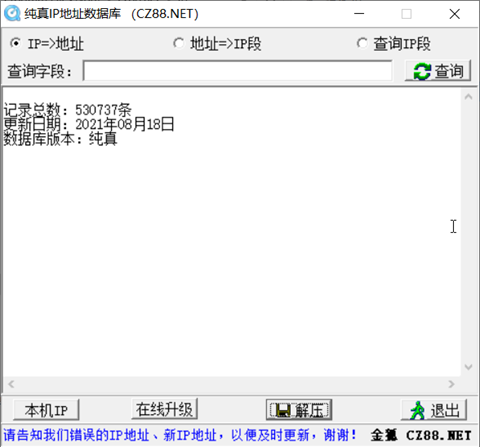文章源自知更鸟-https://zmingcx.com/analysis-of-ip-database.html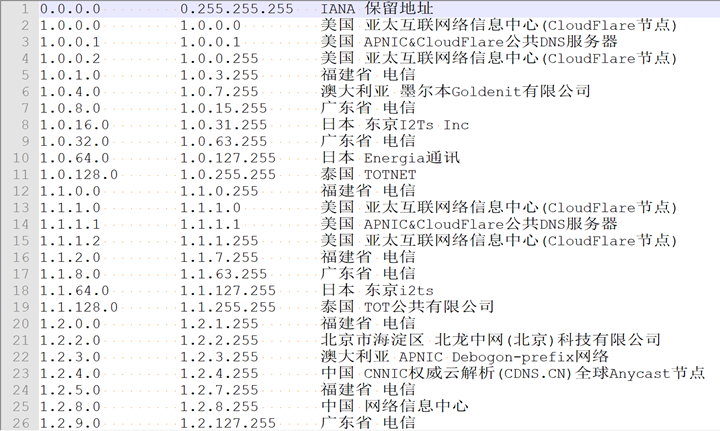文章源自知更鸟-https://zmingcx.com/analysis-of-ip-database.html

## 纯真IP数据库的基本结构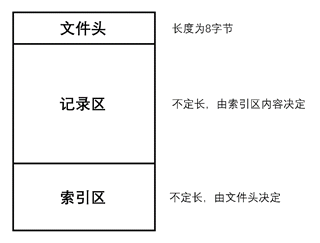文章源自知更鸟-https://zmingcx.com/analysis-of-ip-database.html

### 文件头

QQWry.dat的文件头只有8个字节，其结构非常简单，首四个字节是第一条索引的绝对偏移，后四个字节是最后一条索引的绝对偏移。文章源自知更鸟-https://zmingcx.com/analysis-of-ip-database.html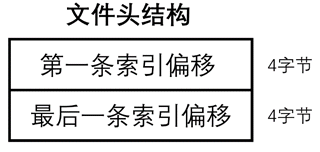文章源自知更鸟-https://zmingcx.com/analysis-of-ip-database.html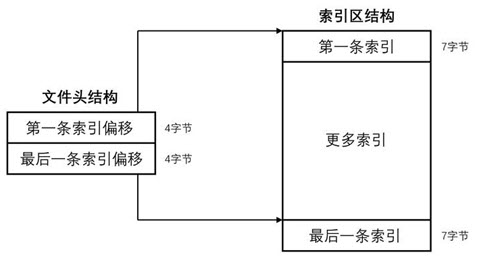文章源自知更鸟-https://zmingcx.com/analysis-of-ip-database.html

### 索引区文章源自知更鸟-https://zmingcx.com/analysis-of-ip-database.html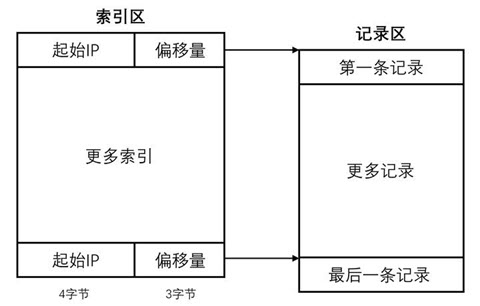文章源自知更鸟-https://zmingcx.com/analysis-of-ip-database.html

### 记录区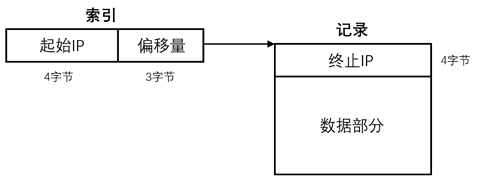文章源自知更鸟-https://zmingcx.com/analysis-of-ip-database.html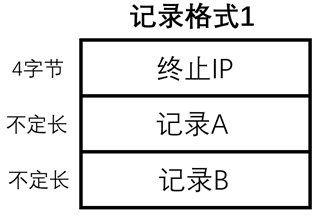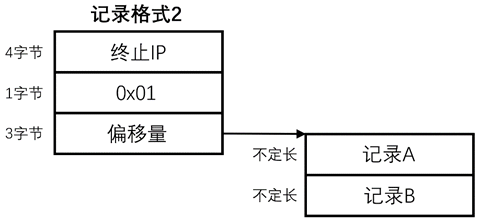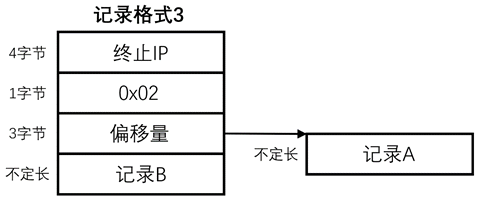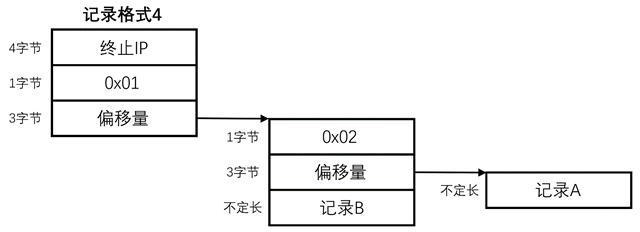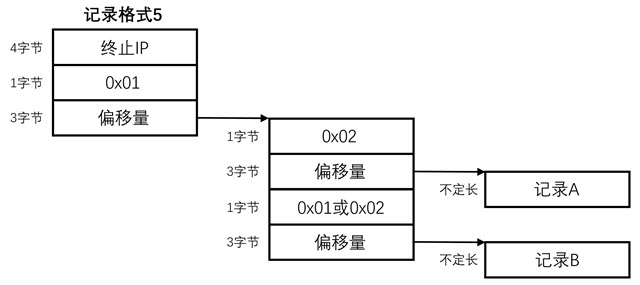## 纯真IP数据库解析示例

Java实现

```/**
* 给定一个ip国家地区记录的偏移，返回一个IPLocation结构
* @param offset 国家记录的起始偏移
* @return IPLocation对象
*/
private IPLocation getIPLocation(long offset) {
try {
// 跳过4字节ip
ipFile.seek(offset + 4);
// 读取第一个字节判断是否标志字节
byte b = ipFile.readByte();
if (b == REDIRECT_MODE_1) {
// 读取国家偏移
long countryOffset = readLong3();
// 跳转至偏移处
ipFile.seek(countryOffset);
// 再检查一次标志字节，因为这个时候这个地方仍然可能是个重定向
if (b == REDIRECT_MODE_2) {
ipFile.seek(countryOffset + 4);
} else
// 读取地区标志
} else if (b == REDIRECT_MODE_2) {
loc.area = readArea(offset + 8);
} else {
loc.country = readString(ipFile.getFilePointer() - 1);
}
return loc;
} catch (IOException e) {
return null;
}
}

/**
* 从offset偏移开始解析后面的字节，读出一个地区名
* @param offset 地区记录的起始偏移
* @return 地区名字符串
* @throws IOException 地区名字符串
*/
private String readArea(long offset) throws IOException {
ipFile.seek(offset);
byte b = ipFile.readByte();
if (b == REDIRECT_MODE_1 || b == REDIRECT_MODE_2) {
long areaOffset = readLong3(offset + 1);
if (areaOffset == 0)
return LumaQQ.getString("unknown.area");
else
} else
}

/**
* 从offset位置读取3个字节为一个long，因为java为big-endian格式，所以没办法
* 用了这么一个函数来做转换
* @param offset 整数的起始偏移
* @return 读取的long值，返回-1表示读取文件失败
*/
private long readLong3(long offset) {
long ret = 0;
try {
ipFile.seek(offset);
ret |= (b3 & amp; 0xFF);
ret |= ((b3 & lt; & lt; 8) & amp; 0xFF00);
ret |= ((b3 & lt; & lt; 16) & amp; 0xFF0000);
return ret;
} catch (IOException e) {
return -1;
}
}

/**
* 从当前位置读取3个字节转换成long
* @return 读取的long值，返回-1表示读取文件失败
*/
private long readLong3() {
long ret = 0;
try {
ret |= (b3 & amp; 0xFF);
ret |= ((b3 & lt; & lt; 8) & amp; 0xFF00);
ret |= ((b3 & lt; & lt; 16) & amp; 0xFF0000);
return ret;
} catch (IOException e) {
return -1;
}
}

/**
* 从offset偏移处读取一个以0结束的字符串
* @param offset 字符串起始偏移
* @return 读取的字符串，出错返回空字符串
*/
private String readString(long offset) {
try {
ipFile.seek(offset);
int i;
for (i = 0, buf[i] = ipFile.readByte(); buf[i] != 0; buf[++i] = ipFile.readByte());
if (i != 0)
return Utils.getString(buf, 0, i, "GBK");
} catch (IOException e) {
log.error(e.getMessage());
}
return "";
}```

PHP实现

```<?php
/**
* IP 地理位置查询类
*
* @author 马秉尧
* @version 1.5
* @copyright 2005 CoolCode.CN
*/
class IpLocation
{
/**
* QQWry.Dat文件指针
*
* @var resource
*/
var \$fp;
/**
* 第一条IP记录的偏移地址
*
* @var int
*/
var \$firstip;
/**
* 最后一条IP记录的偏移地址
*
* @var int
*/
var \$lastip;
/**
* IP记录的总条数（不包含版本信息记录）
*
* @var int
*/
var \$totalip;
/**
* 返回读取的长整型数
*
* @access private
* @return int
*/
function getlong()
{
//将读取的little-endian编码的4个字节转化为长整型数
\$result = unpack('Vlong', fread(\$this - &gt;
fp, 4));
return \$result['long'];
}
/**
* 返回读取的3个字节的长整型数
*
* @access private
* @return int
*/
function getlong3()
{
//将读取的little-endian编码的3个字节转化为长整型数
\$result = unpack('Vlong', fread(\$this - &gt;
fp, 3) . chr(0));
return \$result['long'];
}
/**
* 返回压缩后可进行比较的IP地址
*
* @access private
* @param string \$ip
* @return string
*/
function packip(\$ip)
{
// 将IP地址转化为长整型数，如果在PHP5中，IP地址错误，则返回False，
// 这时intval将Flase转化为整数-1，之后压缩成big-endian编码的字符串
return pack('N', intval(ip2long(\$ip)));
}
/**
* 返回读取的字符串
*
* @access private
* @param string \$data
* @return string
*/
function getstring(\$data = "")
{
\$char = fread(\$this - &gt;
fp, 1);
while (ord(\$char) & gt;
0)
{ // 字符串按照C格式保存，以\0结束
\$data .= \$char; // 将读取的字符连接到给定字符串之后
\$char = fread(\$this - &gt;
fp, 1);
}
return \$data;
}
/**
* 返回地区信息
*
* @access private
* @return string
*/
function getarea()
{
\$byte = fread(\$this - &gt;
fp, 1); // 标志字节
switch (ord(\$byte))
{
case 0: // 没有区域信息
\$area = "";
break;
case 1:
case 2: // 标志字节为1或2，表示区域信息被重定向
fseek(\$this - &gt;
fp, \$this - &gt;
getlong3());
\$area = \$this - &gt;
getstring();
break;
default: // 否则，表示区域信息没有被重定向
\$area = \$this - &gt;
getstring(\$byte);
break;
}
return \$area;
}
/**
* 根据所给 IP 地址或域名返回所在地区信息
*
* @access public
* @param string \$ip
* @return array
*/
function getlocation(\$ip)
{
if (!\$this - &gt;
fp) return null; // 如果数据文件没有被正确打开，则直接返回空
\$location['ip'] = gethostbyname(\$ip); // 将输入的域名转化为IP地址
\$ip = \$this - &gt;
packip(\$location['ip']); // 将输入的IP地址转化为可比较的IP地址
// 不合法的IP地址会被转化为255.255.255.255
// 对分搜索
\$l = 0; // 搜索的下边界
\$u = \$this - &gt;
totalip; // 搜索的上边界
\$findip = \$this - &gt;
lastip; // 如果没有找到就返回最后一条IP记录（QQWry.Dat的版本信息）
while (\$l & lt; = \$u)
{ // 当上边界小于下边界时，查找失败
\$i = floor((\$l + \$u) / 2); // 计算近似中间记录
fseek(\$this - &gt;
fp, \$this - &gt;
firstip + \$i * 7);
\$beginip = strrev(fread(\$this - &gt;
fp, 4)); // 获取中间记录的开始IP地址
// strrev函数在这里的作用是将little-endian的压缩IP地址转化为big-endian的格式
// 以便用于比较，后面相同。
if (\$ip & lt;
\$beginip)
{ // 用户的IP小于中间记录的开始IP地址时
\$u = \$i - 1; // 将搜索的上边界修改为中间记录减一
}
else
{
fseek(\$this - &gt;
fp, \$this - &gt;
getlong3());
\$endip = strrev(fread(\$this - &gt;
fp, 4)); // 获取中间记录的结束IP地址
if (\$ip & gt;
\$endip)
{ // 用户的IP大于中间记录的结束IP地址时
\$l = \$i + 1; // 将搜索的下边界修改为中间记录加一
}
else
{ // 用户的IP在中间记录的IP范围内时
\$findip = \$this - &gt;
firstip + \$i * 7;
break; // 则表示找到结果，退出循环
}
}
}
//获取查找到的IP地理位置信息
fseek(\$this - &gt;
fp, \$findip);
\$location['beginip'] = long2ip(\$this - &gt;
getlong()); // 用户IP所在范围的开始地址
\$offset = \$this - &gt;
getlong3();
fseek(\$this - &gt;
fp, \$offset);
\$location['endip'] = long2ip(\$this - &gt;
getlong()); // 用户IP所在范围的结束地址
\$byte = fread(\$this - &gt;
fp, 1); // 标志字节
switch (ord(\$byte))
{
case 1: // 标志字节为1，表示国家和区域信息都被同时重定向
\$countryOffset = \$this - &gt;
getlong3(); // 重定向地址
fseek(\$this - &gt;
fp, \$countryOffset);
\$byte = fread(\$this - &gt;
fp, 1); // 标志字节
switch (ord(\$byte))
{
case 2: // 标志字节为2，表示国家信息又被重定向
fseek(\$this - &gt;
fp, \$this - &gt;
getlong3());
\$location['country'] = \$this - &gt;
getstring();
fseek(\$this - &gt;
fp, \$countryOffset + 4);
\$location['area'] = \$this - &gt;
getarea();
break;
default: // 否则，表示国家信息没有被重定向
\$location['country'] = \$this - &gt;
getstring(\$byte);
\$location['area'] = \$this - &gt;
getarea();
break;
}
break;
case 2: // 标志字节为2，表示国家信息被重定向
fseek(\$this - &gt;
fp, \$this - &gt;
getlong3());
\$location['country'] = \$this - &gt;
getstring();
fseek(\$this - &gt;
fp, \$offset + 8);
\$location['area'] = \$this - &gt;
getarea();
break;
default: // 否则，表示国家信息没有被重定向
\$location['country'] = \$this - &gt;
getstring(\$byte);
\$location['area'] = \$this - &gt;
getarea();
break;
}
if (\$location['country'] == " CZ88.NET")
{ // CZ88.NET表示没有有效信息
\$location['country'] = "未知";
}
if (\$location['area'] == " CZ88.NET")
{
\$location['area'] = "";
}
return \$location;
}
/**
* 构造函数，打开 QQWry.Dat 文件并初始化类中的信息
*
* @param string \$filename
* @return IpLocation
*/
function IpLocation(\$filename = "QQWry.Dat")
{
\$this - &gt;
fp = 0;
if ((\$this - &gt;
fp = @fopen(\$filename, 'rb')) !== false)
{
\$this - &gt;
firstip = \$this - &gt;
getlong();
\$this - &gt;
lastip = \$this - &gt;
getlong();
\$this - &gt;
totalip = (\$this - &gt;
lastip - \$this - &gt;
firstip) / 7;
//注册析构函数，使其在程序执行结束时执行
register_shutdown_function(array(&amp;
\$this,
'_IpLocation'
));
}
}
/**
* 析构函数，用于在页面执行结束后自动关闭打开的文件。
*
*/
function _IpLocation()
{
if (\$this - &gt;
fp)
{
fclose(\$this - &gt;
fp);
}
\$this - &gt;
fp = 0;
}
}```

Python实现：

```#!/usr/bin/env python
# -*- coding: utf-8 -*-
import sys
import socket
from struct import pack, unpack
class IPInfo(object):
def __init__(self, dbname = "qqwry.dat"):
self.dbname = dbname
f = open(dbname, 'rb')
f.close()
(self.firstIndex, self.lastIndex) = unpack('<II', self.img[:8])
self.indexCount = (self.lastIndex - self.firstIndex) / 7 + 1
def getString(self, offset = 0):
o = self.img.find('\0', offset)
return self.img[offset:o]
def getLong3(self, offset = 0):
s = self.img[offset: offset + 3] + '\0'
return unpack('<I', s)
def gbk2utf8(self, str):
return unicode(str, 'gbk').encode('utf-8')
def utf82gbk(self, str):
return unicode(str, 'utf-8').encode('gbk')
def find(self, ip):
low = 0
high = self.indexCount
while (low < high - 1):
mid = low + (high - low) / 2
o = self.firstIndex + mid * 7
start_ip = unpack('<I', self.img[o: o+4])
if ip < start_ip:
high = mid
else:
low = mid
return low
img = self.img
byte = ord(img[offset])
if byte == 1:
offset = self.getLong3(offset + 1)
byte = ord(img[offset])
if byte == 2:
zone = self.getString(self.getLong3(offset + 1))
offset += 4
else:
zone = self.getString(offset)
offset += len(zone) + 1
byte = ord(img[offset])
if byte == 2:
area = self.getString(self.getLong3(offset + 1))
else:
area = self.getString(offset)
return (zone, area)
ip = unpack('!I', socket.inet_aton(ip))
i = self.find(ip)
offset = self.firstIndex + i * 7
offset = set.getLong3(offset + 4)
return self.getAddr(offset + 4)
def main():
i = IPInfo()
ip = sys.argv
(zone, area) = i.getIPAddr(ip)
print '%s %s/%s' % (ip, zone, area)
if __name__ == '__main__':
main()```•小蜜 0

市场上有许多免费的数据库，也提供下载，精准度也ok，你可以试试看 https://lite.ip2location.com

•轨魅网 2

收藏